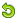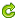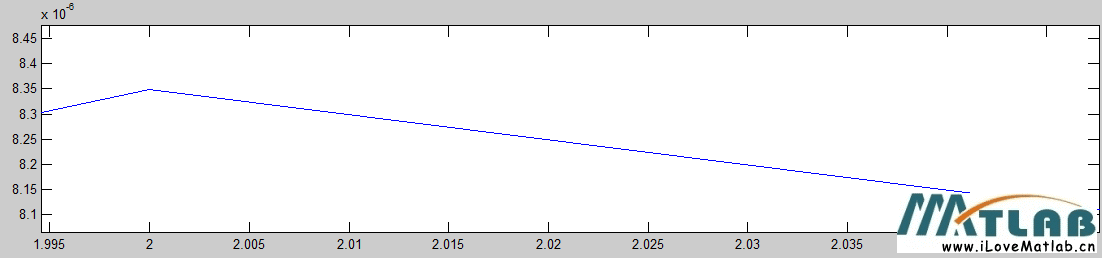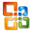• 语音写出（wavwrite）

千次阅读 2021-12-04 18:23:59
wavwrite (y,“文件名”) wavwrite (y, Fs,“文件名”) wavwrite (y, Fs, N,“文件名”) 描述 wavwrite支持多通道WAVE数据，每个样本高达32位，并支持写入24和32位。wav文件。 wavwrite(y，‘filename’)写入由字符...

编写Microsoft WAVE (.wav)声音文件

语法
wavwrite (y,“文件名”)
wavwrite (y, Fs,“文件名”)
wavwrite (y, Fs, N,“文件名”)

描述
wavwrite支持多通道WAVE数据，每个样本高达32位，并支持写入24和32位。wav文件。

wavwrite(y，‘filename’)写入由字符串filename指定的WAVE文件。 数据应按每列一个通道排列。 在[-1，+1]范围外的振幅值在写入之前被剪切。

wavwrite(y,Fs，‘filename’)指定数据的采样速率Fs，以赫兹为单位。

wavwrite(y,Fs,N，‘filename’)强制写入一个N位的文件格式，其中N <= 32。

展开全文语音识别 人工智能 wavwrite matlab wav
• 本帖最后由 zhh2100220115 于 2014-12-19 13:20...还是仅仅这里wavwrite函数的问题。总之做了些试验，还是用和原来差不多的程序：close allclcclear allt = 1;fs = 100;n = 0:1/fs:t-1/fs;f1 = 10; A = 0.25;x = A*s...

本帖最后由 zhh2100220115 于 2014-12-19 13:20 编辑

还在想那个误差的问题呢。在想是否涉及到浮点数和整型数之间转换时的误差分析问题。还是仅仅这里wavwrite函数的问题。总之做了些试验，还是用和原来差不多的程序：

close all

clc

clear all

t = 1;

fs = 100;

n = 0:1/fs:t-1/fs;

f1 = 10; A = 0.25;

x = A*sin(2*pi*f1*n);

subplot(211)

plot(x,'r')

hold on

N = 24;

wavwrite(x,fs,N,'test.wav');     %MATLAB 写wav文件，看最大最小值

[y, Fs, nbits] = wavread('test.wav');   % MATLAB读wav文件，看最大最小值，预计是在-1和1之间

plot(y,'g')

Amp = num2str(A);bits = num2str(N);

title(strcat('A=',Amp,' N=',bits));

hold off

z = x - y';

subplot(212)

plot(z)

我本来想，A的值在0到1之间，按照前面版主的分析，应该存在很小的误差，基本应该是很准的了。结果也确实如此，都是很小的数量级。

依然，本来想，对于同一个N值，误差是不是就是定了呢？比如，int16，是不是最小值的一半就是误差精度呢？也就是int16对应的误差最大值是：      1/(2^15-1)/2 = 1.525925473799860e-05

可是，试验不同的A值，得到的误差的最大值(从作差图上的峰值放大看)却是不同的！详见附件1。

变换不同的A(0

看来原来猜测不对。那么，会不会是这样量化的呢——将振幅当成最大值，那么精度就和振幅有关了？如，A=0.5，int16的计算为：0.5/(2^15-1)/2=7.629627368999298e-06

放大相关图像，得到A=0.5时误差最大值为：8.34e-6.见附图。

可见，这种猜测的误差最大值和实际的还是有些差距的，而且不可解释的是居然实际的会大，上面分析的可是按照误差最大值来的！这是为什么呢？猜测又不对了么？

同理，当A不变时，对于不同的N，误差最大值貌似不存在倍数关系。这些比值是比较奇怪的数。详见附件2.

当A改变，N改变时，误差最大值之比更无法找到一些比较固定的变化趋势。见附件2.相应程序为：

close all

clc

clear all

t = 1;

fs = 100;

n = 0:1/fs:t-1/fs;

f1 = 10; A = 0.25;

x = A*sin(2*pi*f1*n);

subplot(221)

plot(x,'r')

hold on

N1 = 8;

wavwrite(x,fs,N1,'test.wav');

plot(y,'g')

Amp1 = num2str(A);bits1 = num2str(N1);

title(strcat('A=',Amp1,' N=',bits1));

hold off

z1 = x - y';

max1 = max(max(z1));

subplot(222)

plot(z1)

title(' x - y'' error')

N2 = 24;

wavwrite(x,fs,N2,'test.wav');     %MATLAB 写wav文件，看最大最小值

[y, Fs, nbits] = wavread('test.wav');   % MATLAB读wav文件，看最大最小值，预计是在-1和1之间

subplot(223)

plot(x,'r')

hold on

plot(y,'g')

Amp = num2str(A);bits2 = num2str(N2);

title(strcat('A=',Amp,' N=',bits2));

hold off

z2 = x - y';

max2 = max(max(z2));

% figure(2)

subplot(224)

plot(z2)

precision_quotient = max1/max2;

title(strcat(' When A=',Amp1,',then:',bits1,'/',bits2,' bits precision quotient is ',num2str(precision_quotient)));

不知道该怎么解释这些奇怪的数了。

1.png

(23.32 KB, 下载次数: 0)

2014-12-19 12:43 上传12014-12-19 12:43 上传

点击文件名下载附件

82.01 KB, 下载次数: 0

附件12014-12-19 12:44 上传

点击文件名下载附件

257.01 KB, 下载次数: 0

附件2

展开全文• wavwrite(y,filename) wavwrite(y,Fs,filename) wavwrite(y,Fs,N,filename) audiowrite - Write audio file This MATLAB function writes a matrix of audio data, y, with sample rate Fs to a file called ...

This MATLAB function loads a WAVE file specified by the string filename,

returning the sampled data in y.

[y, Fs, nbits, opts] = wavread(filename)

This MATLAB function reads data from the file named filename, and returns

sampled data,y, and a sample rate for that data, Fs.

wavwrite - Write WAVE (.wav) sound file

This MATLAB function writes the data stored in the variable y to a WAVE file

called filename.

wavwrite(y,filename)

wavwrite(y,Fs,filename)

wavwrite(y,Fs,N,filename)

audiowrite - Write audio file

This MATLAB function writes a matrix of audio data, y, with sample rate Fs to a

file called filename.

audiowrite(filename,y,Fs)

audiowrite(filename,y,Fs,Name,Value)

filename = 'ISM.wav';

wavwrite(y,Fs,filename);

audiowrite(filename,y,Fs);

以上是wavwrite函数，audiowrite函数区别

展开全文• 前几天群里有人提出一个问题：MATLAB里，同样频率的信号写入/读取，为什么频率感觉不同？ 测试code: fs = 2000; f0 = 20; t = 0:1/fs:1; subplot 211 ...wavwrite(signal,fs,'test.wav'); data = ...

前几天群里有人提出一个问题：MATLAB里，同样频率的信号写入/读取，为什么频率感觉不同？

测试code:

fs = 2000;
f0 = 20;
t = 0:1/fs:1;
subplot 211
signal = sin(2*pi*t*f0);
plot(t,signal);hold on;
wavwrite(signal,fs,'test.wav');
plot(t,data,'r--');

subplot 212
signal = 3*sin(2*pi*t*f0);
plot(t,signal);hold on;
wavwrite(signal,fs,'test.wav');
plot(t,data,'r--');

结果：幅度放大之后，信号失真，也难怪频率听起来不同了。看一看wavwrite特性：为了让信号不失真，可以采取两个办法：1）信号归一化;2)利用NBITs=32设置：修改：

wavwrite(signal,fs,32,'test.wav');

转载于:https://www.cnblogs.com/xingshansi/p/6884220.html

展开全文• sound,wavwrite函数的使用总结

万次阅读 2016-03-24 11:36:35
一、sound函数的使用 1.功能：将数据变成声音播放 2.使用方法：（1）sound(y,Fs)  （2）sound(y)  （3）sound(y,Fs,bits) 3.注意：（1）数据y范围在[-1，+1] ... （2）不写Fs，默认采样率为 8192...二、wavwritematlab sound wavwrite 函数 数据
• 课程设计数字信号处理 说明书 设计题目基于MATLAB的简单音乐合成 姓 名 专业年级 学 号 指导老师 时 间 2015年6月25日 数字信号处理课程设计任务书 题目 基于MATLAB的简单音乐合成 主要 内容 1自学Matlab软件了解...
• audiowrite(filename,y,Fs)audiowrite(filename,y,Fs,Name,Value)二，解释注意：在新版本的matlab中不再使用wavwrite，推荐使用audiowritefilename指想要保存文件的文件名，一般为想要取得名字，比如想生成音频文件...
•  % 计算ICA后的矩阵 % 将混合矩阵重新排列并输出 subplot(3,2,5),plot(ICAedS(1,:)),title('ICA解混声音1'), subplot(3,2,6),plot(ICAedS(2,:)),title('ICA解混声音2'), wavwrite(ICAedS(1,:),Fs1,'SS1.wav');...matlab网上求助
• matlab开发的wav播放器，可以实现播放、文件路径选取等基本功能。matlab
• 注意：在新版本的matlab中不再使用wavwrite，推荐使用audiowrite 函数语法 wavwrite(y,filename) 将保存在变量y的数据,保存到wav文件filename中，默认的采样率为8000Hz，采样位数为16位，y的各列为各个通道，...
• 处理后的数据如是音频数据，则可用wavwrite转换成WAV格式文件或用sound、wavplay等函数直接回放。下面分别介绍MATLAB在音量标准化、声道分离合并与组合、数字滤波、数据转换等音频信号处理方面的技术实现。 　 　...语音信号处理
• wavwrite(allData(513:end)./32768, fs, file); %SPHERE 文件头1024字节 end 第三段代码：check_wav.m，这个函数主要用来检查所有文件是否已经正确转换完成。 clear all; files = find_wav('E:\TIMIT'); for ...语音识别 matlab
• 如何用matlab对采集的wav信号做FFT运算关注:133答案:2mip版解决时间 2021-01-28 17:08提问者阳光在浪尖跳动2021-01-27 17:21如何用matlab对...y = wavread(filename) 将信号幅度减半后， 存波形：wavwrite(y,filen...
• Timit SPHERE格式转换

千次阅读 2017-07-18 10:12:18
wavwrite(allData(513:end)./32768, fs, newfile_path); %SPHERE 文件头1024字节   % fclose(fileID); end 3、check_wav.m clear all; files = find_wav('.'); for fileIdx = 1:length(files) ...Timit SPHERE
• 答：输出波形文件和退出系统 这个功能由输出文件按钮来完成，是应用MATLAB的wavwrite函数将音频信号转换成.wav文件，文件中也包含了采样频率和数据宽度选项，增加此项的目的是为了能让更专业的音频处理软件对信号...matlab
• 用Matlab录制、读取音频

万次阅读 2018-01-10 20:41:20
wavwrite函数已经停用，所以在下面讲解一下用来替换的audiowrite函数 这个audiowrite()函数也有两种调用方法： audiowrite(filename,y,Fs) audiowrite(filename,y,Fs,Name,Value) 给大家解释下，filename...
• Python--读取wav格式文件(2013-05-28 06:56:22)标签：格式文件读取杂谈1、import wave 用于读写wav文件它提供了一个方便的WAV#26684;式接口。但是不支持压缩/解压缩，支持单声道/立体声。读取#26684;...
• 在读取文件时用Qfile file.read先读取表头信息再读取音频数据 ... 数据滤波处理时使用计算出滤波参数后，在线性卷积时发现总会卡死到双重for循环中（下面是已经修改后的） for( int i=0; i<...QT wav
• matlab 未定义输入参数相对应的函数 Optimization running. Error running optimization. 未定义与 ‘double’ 类型的输入参数相对应的函数 ‘f’。 可能问题：当前工作路径和函数的路径不对应 ...matlab
• 语音处理中，都是讲语音信号分解成时域范围内波形，或者频域范围内的图。比如经过傅里叶变换生成的频谱图。那么加入我们知道一堆的声音数据，我们怎么将它们还原成规定的音频格式呢，可以是mp3，wav等等的音频格式。...matlab 傅里叶变换 傅里叶 audio 函数
• Octave 是一个 Linux 上类似于 Matlab 的软件，它拥有数量众多的函数和命令，支持声音采集、记录、回放以及音频信号的数字化处理，用于娱乐应用、研究、医学以及其它科学领域。在本教程中，我们会在 Ubuntu 上使用 ...
• wavwrite和wavappend命令来读取，写入和附加WAV文件。 这是一个使您入门的示例。 它生成一些数据，将其写入文件，然后读回数据。 wavplay还提供了简单的音频播放。 julia> using WAV julia> x = [0:7999;] julia> y ......